Xmountains is a fractal terrain generator written by Stephen Booth This document describes what it does and how it works.

The basic idea behind a fractal landscape is to generate a continuous surface which varies in height randomly but with the random variation obeying a particular statistical law.

In the case of a fractal landscape the average difference in height between pairs of points separated by a distance l should go as a power law in l. If you are only interested in random terrain generation rather than fractal self-similarity then you could use other functions of l that tend to zero as l tends to zero. If the function does not tend to zero then the result will not be continuous. I use the following definition:

```                      2H
<|H1 - H2|>  =  l
```

For values of H below 0.5 as l gets small the variation in height is greater than the horizontal distance between the points (l) so the surface becomes space-filling. For values of H at 0.5 or above the surface becomes smoother as H increases. This is because the variation in height at short length scales is a smaller fraction of the variation at longer length scales. When displaying the surface you usually re-scale the whole lot by a constant factor so it is the relative variation that matters.

This program uses a modified form the mid-point displacement algorithm

The mid-point displacement algorithm is a recursive algorithm. Starting from a crude outline of the surface more and more detail is added at progressively smaller length scales. Each iteration doubles the resolution of a 2 dimensional grid of altitudes.

This is done in 2 stages:

```A           B                  A           B
stage1
--------->            E

C           D                  C           D

A           B                  A     F     B
stage2
E         -------->      G     E     H

C           D                  C     I     D
```

More detail can then be filled in by recursion:

```A     F     B                  A     F     B
stage1           *     *
G     E     H   -------->      G     E     H
*     *
C     I     D                  C     I     D

A     F     B                  A  +  F  +  B
*     *       stage2        +  *  +  *  +
G     E     H   -------->      G  +  E  +  H
*     *                     +  *  +  *  +
C     I     D                  C  +  I  +  D

```

The new points are generated by taking an average of the surrounding points and adding a random offset. In order to generate the power law behaviour described above the random offset is scaled by a factor proportional to a power (2H in my notation) of the distance between the new points and the surrounding points. The results seem to be a little better if the random offsets are taken from a gaussian distribution. The basic idea is very similar to that used by the Koch snowflake.

The modifications to the standard algorithm are as follows: There are three optional regeneration steps to reduce creasing. These are controlled by the -s flag.

The -x flag (cross update) controls whether the midpoints (E) are included in the average when performing the stage2 update or if only the corner points are used.

## Creasing

When I started in this game "creasing" was a big problem with square grid mid-point displacement surfaces and explicit smoothing steps were introduced to get rid of these. You can make my program revert to these early algorithms by specifying "-s 0" Things are particularly bad if you calculate the middle of the sides independently of the middle of the square ("-x" in my program) This was not uncommon in the other programs I have seen.

If you only use a pair of points to perform the average for the middle of the sides then for any point that lies on one of the sides of a large length scale square its value depends ONLY on the values of other points that lie along that side. This line then forms a crease. Because no information ever crosses this line it acts as a kind of "event horizon". The heights of points on the crease are calculated independently of everything else and then the surfaces on the 2 sides form to match this line but the 2 surfaces are independent of each other. The crease is a mis-match between the 2 independent surfaces that are only constrained to follow a common boundary that has been specified independently of each of them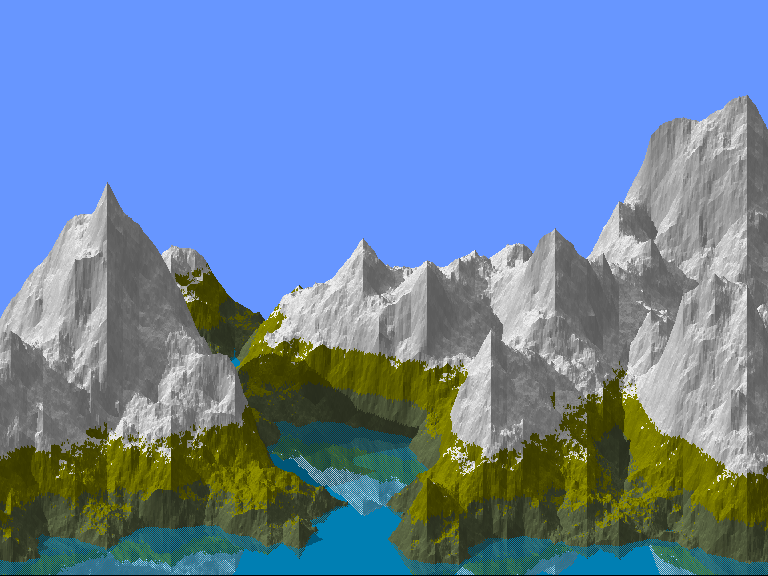When you split the update into 2 steps:

1. generate the middle of the square with an average of the 4 corners and a random offset based on a length scale of L/sqrt(2).
2. generate the sides as an average over 4 points (2 corner points and 2 middle of square points) followed by a random offset based on a length scale of L/2.
the information flows sideways into the sides of the large squares. The problem does not go away but instead of creases along the sides of the squares you get conical deformations at the corners of the squares: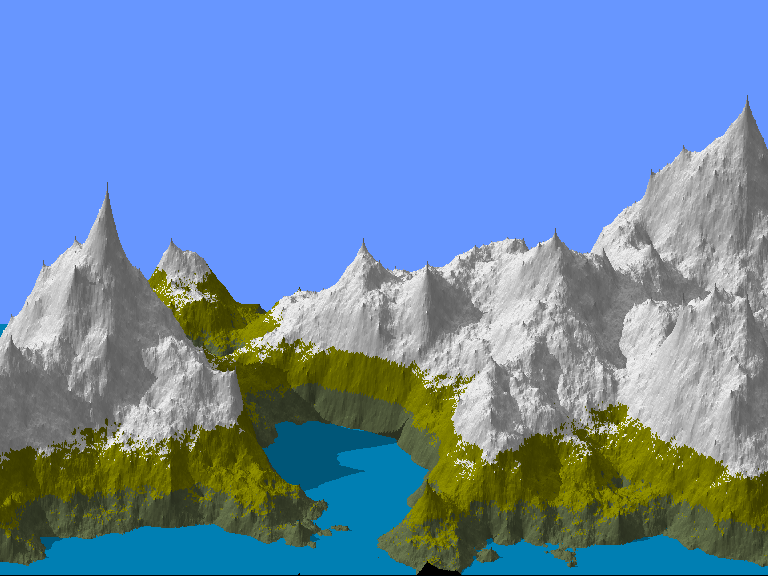This is an improvement but not the whole story.

### Regeneration steps

The root cause of creasing and the conical deformations is that the position of some points are fixed by the early (long-distance) iterations of the fractal algorithm. We are trying to generate a surface where the average difference in height between points on the surface is a function of the distance between them. Ideally two points should have an equal effect on each other. Instead we have implemented a hierarchy of points where the information only flows in one direction.

The solution I came up with was to use the early iteration to set the overall trend of the surface rather than setting the exact position of particular points. This is done by a 2 steps forward/1 step back method. In the original algorithm the results at the end of one iteration are used to generate a new set of points with each iteration adding detail at a length scale of half the previous iteration. I added a second stage to each iteration that consisted of discarding all of the points generated by previous iterations and regenerating them from the new points. I think this is a lot closer to the desired result because every point is the result of updates at all length scales.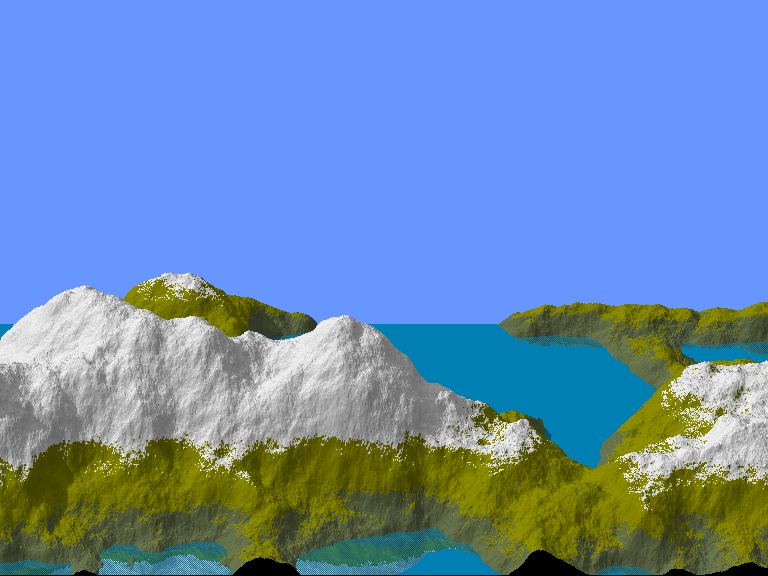However I have a suspicion that it may result in a slight shift in the fractal dimension. It would be nice to measure the fractal dimension produced as a function of the input parameter to the algorithm.

A regeneration step recalculates the height of existing points using an average and offset from a newer generation of points. In xmountains the three regeneration steps are:

Step 1
recalculate corner points (A,B,C,D) from the midpoints (E) after the stage1 update.
Step 2
recalculate midpoints (E) from the edge points (F,G,H,I) after the stage2 update
Step 3
recalculate corner points (A,B,C,D) from the edge points (F,G,H,I) after the stage2 update

The regeneration stages are turned on by the smoothing parameter (-s flag)

```  flag	Step-3	Step-2	Step-1
0	off	off	off
1	on	off	off
2	off	on	off
3	on	on	off
4	off	off	on
5	on	off	on
6	off	on	on
7	on	on	on
```

The default is to just use step 3 (-s 1)

When performing the regeneration steps the random offset is added to an average of the new points.

I came across a different solution in the xmnts program by Paul Sharpe @ DEC, Reading, England. who also modified the old points at each iteration, but he only added an additional random offset to the old points.

I added this capability to my program. The -X and -Y parameters trade off the two methods if you leave them at 0.0 (the default) you get my algorithm. If you set them to 1.0 you get Paul's and values in-between give you a mixture of the two, i.e. a weighted average of the new points and the old value of the point.

### How well does this all work

The relative importance of the different algorithms can easily be seen if we remove the random element from the algorithm and replace the gaussian random number with a constant value. Without any random variation we should get a completely smooth surface. Without any regeneration steps or cross updates we get a surface consisting only of creases.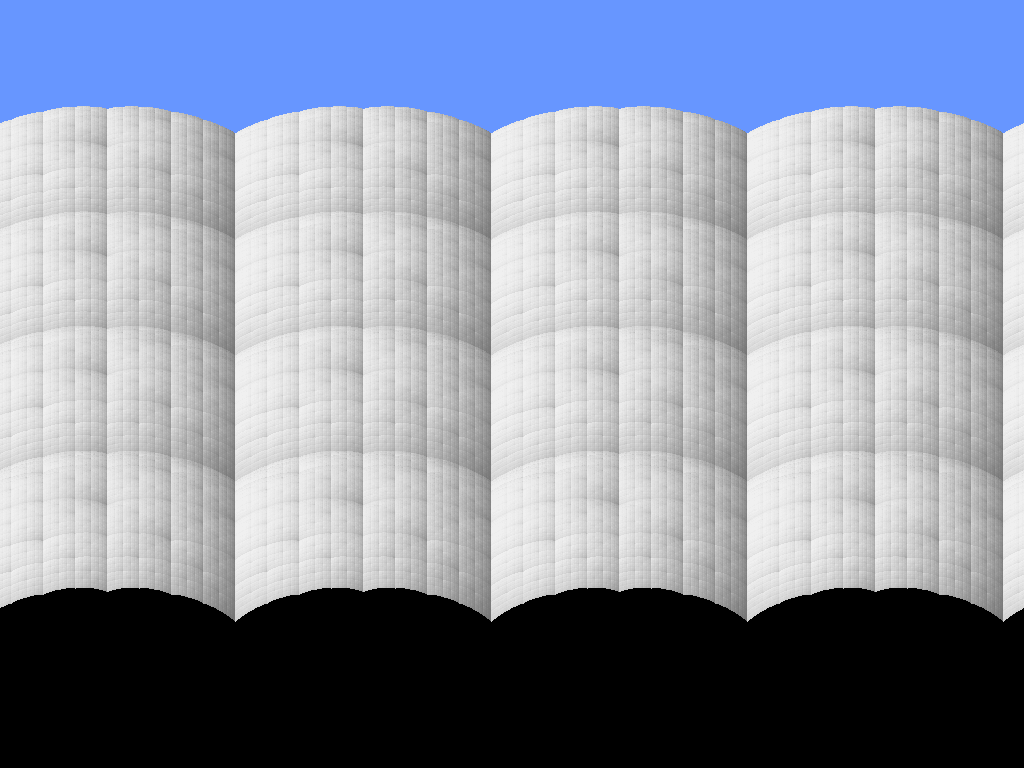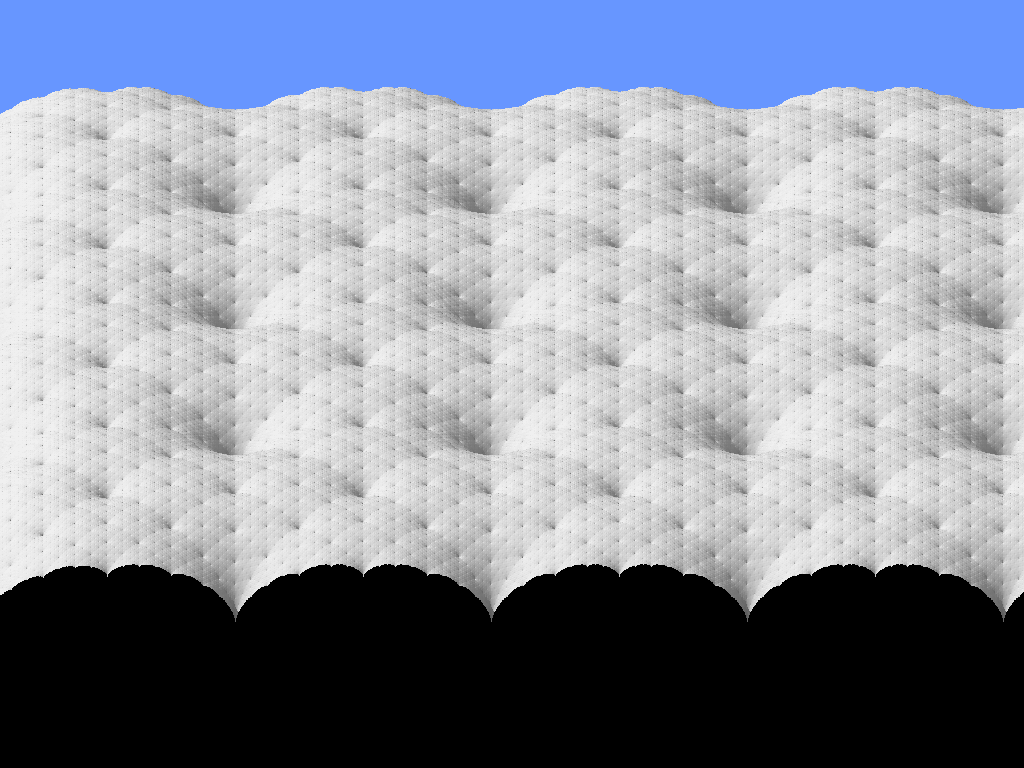This is an improvement as the surface has better rotational symmetry but it still has significant artifacts. These are completely missing when regeneration steps are used.

## Pipelining

The other trick I came up with was to pipeline the code. This is also responsible for much of the speed because it reduces swapping and improves cache use. I don't think that anyone else does this but the idea is straightforward once you think of it.

If you think of the normal algorithm it is possible to add extra detail to a region of the surface without adding this level of detail to the entire surface. Each iteration of the algorithm increases the number of squares by 4. You can then add further detail to a restricted region of the surface by only applying the next level of recursion to a subset of these squares. Obviously some squares you choose not to update will end up partially updated because they share sides with updated squares.

As an aside this selective updating allows you to zoom-in on a surface efficiently. You don't bother updating squares outside of your field of view and you keep adding more detail to the squares you can see until the sides of the smallest level squares subtends less than a maximum angle at your point of view (i.e. the sides always look short).

What I do in xmountains is slightly different. I only perform updates necessary to fully update the left hand row of squares. These fully updated points are used to generate a column of pixels in the output and then discarded. The new left hand edge is not fully updated so additional updates are now required. Imagine I have a level-1 surface as follows:

```1   1   1

1   1   1

1   1   1

```
And that I have some method of generating new points on the right hand side of this surface (I will show how I do this later but for the time being assume this is the top iteration level and we can generate new points by applying random offsets to a constant value).

I can use these points to fill in part of the left hand squares

```1 2 1   1
2 2
1 2 1   1
2 2
1 2 1   1

```
Now scroll everything to the left
```1   1

1   1

1   1

```
and add in a new third column on the right hand side
```1   1   1

1   1   1

1   1   1

```
This returns us to our original state. This process can be repeated indefinitely. For each column of points it inputs on the right it outputs 2 columns on the left with level of detail doubled. If you want to perform N levels of update then you chain together N of these steps. If you want to use a more complicated update (For example the regeneration steps and the cross update) then the same basic approach applies but the details get more complicated. In the xmountains the equivalent to the step described above is a routine called next_strip.

## xmountain command line flags

```xmountains: version 2.3
usage: xmountains -[bqgdPEmMrBZIASFTCapcevfRltxsXYH]
-b       [false] use root window
-q       [false] reset root window on exit
-g string     window geometry
-d string     display
-P filename   write PID to file
-E       [false] toggle explicit expose events
-m       [false] print map
-M       [false] implement reflections
-r int    # columns before scrolling
-B int    # shades in a colour band
-n int    # number of colours
-Z int    time to sleep before scrolling
-I float [40.000000] vertical angle of light
-A float [0.000000] horizontal angle of light
-S float [0.600000] vertical stretch
-T float [0.500000] vertical shift
-W float [0.000000] sealevel
-F int    reduce variation in the foreground
-G float [-1.000000] average foreground height
-C float [0.300000] contour parameter
-a float [2.500000] altitude of viewpoint
-p float [4.000000] distance of viewpoint
-c float [1.000000] contrast
-e float [0.300000] ambient light level
-v float [0.600000] vertical light level
Fractal options:
-f float [0.650000] fractal dimension
-R int    rng seed, read clock if 0
-l int    # levels of recursion
-t int    # non fractal iterations
-x       [true] cross update
-s        smoothing (0-7)
-X float [0.000000] fraction of old value for rg2 & rg3
-Y float [0.000000] fraction of old value for rg1
-H            print short description of algorithm.
```

## X window graphics

The algorithms and code for xmountains were originally developed for to drive particular graphics hardware and then ported to the X-window system. This history can still be seen in the following ways
• All the options are given as command line flags.
• The code assume a CLUT based colour model rather than direct RGB values.
Most of the suggestions I get for changes to xmountains involve either adding a X control panel to set parameters or implementing 24-bit graphics. Actually neither of these makes a great deal of sense.

To save memory the program does not retain any knowledge of currently displayed surface. The only information that is retained is the final bitmap image. This makes it very difficult to change the viewpoint while the program is running (though you could probably get away with slow gradual changes).

The program can already utilise 24-bit colour displays the default parameters are set to use less than 256 colours but the -B or -n flags can be used to increase the number of colours. The quality of the final image remains pretty much the same though because there are already 80 shades of each base colour. If you only ever run on a 24-bit display you may want to try increasing the number of base colours by increasing N_BANDS in paint.h and modifying the set_clut routine in artist.c